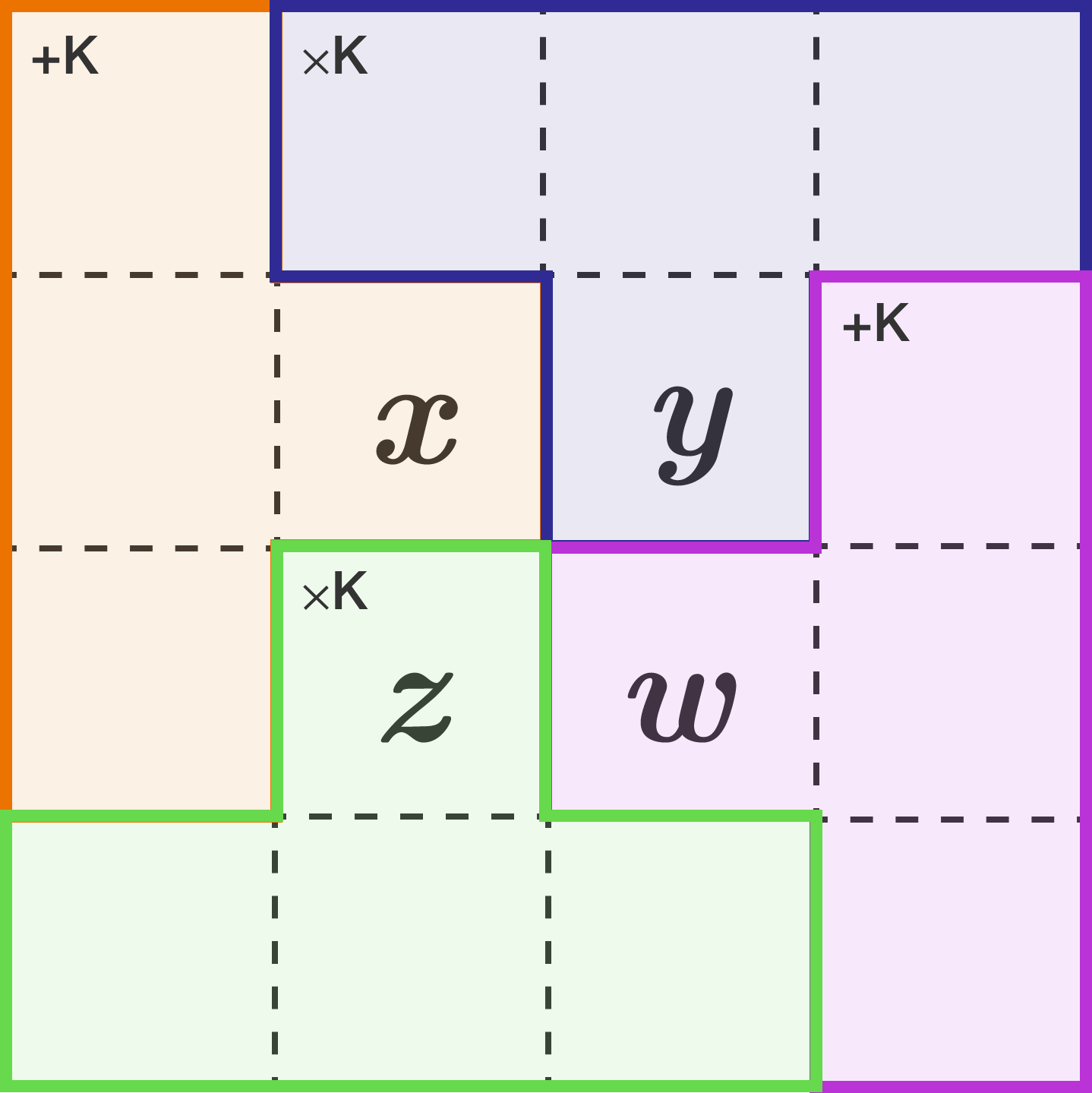# $T$-Tetrominoes KenKen Puzzle!4-by-4 puzzle, consisting of 4 Tetrominoes!

The following are the rules of the $4\times 4$ Kenken above:

• Each row contains exactly one of each digit 1 through 4.
• Each column contains exactly one of each digit 1 through 4.
• Each colored group of cells is a cage containing digits which achieve the result $(K)$ using the specified operation $(+$ or $\times)$.

First, solve the puzzle, where $K$ is a strictly positive integer.

If there is a unique value of $K$, determine whether or not $x + y + z + w = K$.

Otherwise, i.e. if you believe there are more than one solutions with different values of $K$ for the given puzzle, choose "Multiple solutions."


Note: Unlike standard Sudoku, KenKen can have cage(s) with repeated digits.

Hint: Observe the structure of this puzzle carefully. What can be said about those tetrominoes and the numbers in each row and column?

×